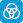Close message Scootle will stop supporting resources that use the Adobe Flash plug-in from 18 Dec 2020. Learning paths that include these resources will have alerts to notify teachers and students that one or more of the resources will be unavailable. Click here for more info.

# Mathematics / Year 10 / Number and Algebra / Linear and non-linear relationships

Curriculum content descriptions

Solve linear equations involving simple algebraic fractions (ACMNA240)

Elaborations
• solving a wide range of linear equations, including those involving one or two simple algebraic fractions, and checking solutions by substitution
• representing word problems, including those involving fractions, as equations and solving them to answer the question
General capabilities
• Numeracy Numeracy
ScOT terms

Simple fractions,  Linear equations,  Algebraic terms

## Refine results by

Year level 9-10
Resource type
Learning area Mathematics

## Refine by topic

Related topic### Linear equations and inequalities

This resource is a collection of screen displays of questions relating to linear equations and inequations. Graphing tasks involve determining the coordinates of two points on the linear function. Other tasks are presented as multiple-choice questions and involve finding slopes of lines, calculating intercepts with the ...### Secondary mathematics: different representations

These seven learning activities, which focus on 'representations' using a variety of tools (software) and devices (hardware), illustrate the ways in which content, pedagogy and technology can be successfully and effectively integrated in order to promote learning. In the activities, teachers use different representations ...### The Numberline

An interactive tool that can help students explore a number line, including points representing integers, fractions and decimals### Trade and Investment at a Glance

Using an illustrated report from the Department of Foreign Affairs and Trade, this Teacher guide provides ten learning sequences that engage students in the analysis and interpretation of data about Australian imports and exports. Students: identify Australia's major exports and imports; investigate international trade ...### Using linear equations to model population growth, Hooke's Law and parcel shipping charges

In this animated presentation students are provided with three examples of mathematical modelling in real-world settings. Linear functions are used to model population growth, Hooke's Law and parcel shipping charges.### BBC Bitesize: more on equations - revision

These illustrated information sheets revise the methods of solving linear equations from simple ones with one unknown term to ones with the unknown on both sides. The solution of equations containing brackets or fractions is also considered. Full algebraic working is shown for sample equations. Students solve equations ...### reSolve: Algebra: Think of a number- Binomial Equations

This lesson engages students in investigating a 'think of a number' game and then model it visually and algebraically. This develops skills in algebraic operations including expanding, factorising and collecting like terms. Students investigate whether the game will work for any number and are challenged to generate the ...### BBC Bitesize: graphs - revision

These illustrated information sheets revise the rules for vertical and horizontal lines and the meaning of m and c in the rule y = mx + c. Students find the rule for a given linear graph. Tables of values are created so that graphs can be plotted. This resource is one of a series of online resources from the BBC's Bitesize ...### TIMES Module 33: Number and Algebra: factorisation - teacher guide

This is a 17-page guide for teachers. It continues the discussion of factorisation. In particular, the techniques for the factorisation of quadratic expressions are presented.### TIMES Module 36: Number and Algebra: formulas - teacher guide

This is a 13-page guide for teachers. It introduces the use of formulas, and includes substitution and the solution of the resulting equations.### TIMES Module 34: Number and Algebra: quadratic equations - teacher guide

This is a 19-page guide for teachers. It introduces quadratic equations and methods for solving them.### Data representation and interpretation using scatterplots - teacher resource

This resource is a professional learning package designed for pre-service teachers. It demonstrates and investigates the interrelated content, pedagogy and technology knowledge used in a series of mathematics lessons that focus on looking for a relationship between two variables. The resource provides video footage of a ...### Desmos Graphing Calculator - Google Play app

Plot functions, create tables, add sliders and animate your graphs. Touch points of interest on the graph to show maximums, minimums and points of intersection. Type in an equation and watch the calculator solve the problem. Free when reviewed on 12/5/2015.### Graphing: line of best fit

Plot some data points on a graph. Estimate the position for a line of best fit. Use a graphing tool to check your estimate. Add more points to see how they affect the position of the line of best fit.### Green growth indicators

This is a web resource consisting of a database of selected indicators for monitoring progress towards green growth in OECD countries, including Australia, and BRIICS economies - Brazil, Russian Federation, India, Indonesia, China and South Africa - from 1990 to 2012. The indicators are based on environmental and resource ...### Measuring Millennium Development Goals progress

This is a unit of work about progress towards achieving the United Nation's Millennium Development Goals (MDG). Written from a global education perspective, the resource consists of four single- or multi-lesson activities, titled: What do the Millennium Development Goals measure?; Tracking the world's progress; Comparing ...### Graphing calculator by Desmos - iTunes app

Plot functions, create tables, add sliders and animate your graphs. Touch points of interest on the graph to show maximums, minimums and points of intersection. Type in an equation and watch the calculator solve the problem. Free when reviewed on 12/5/2015.